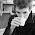## Monday, November 11, 2013

### A problem on Reciprocals

(I thought I had posted this a while back but I can't find it in past blogs
so I think I did not. I DID post a diff problem on reciprocals.)

Here is the question I graded a while back on a  Maryland Math Olympiad.
I request that you do it and post your answer as a comment- I'll be curious
I will post the solutions the students used in my next post and comments
on how they were similar or different than yours.
The students had two hours to do five problems.
This was problem 2.

The equalities 1/2 + 1/3 + 1/6 = 1 and 1/2 + 1/3 + 1/7 + 1/42 = 1
express 1 as a sum of three (resp. four) reciprocals.

PART A: Find five distinct positive integers a,b,c,d,e  such that

1/a + 1/b + 1/c + 1/d + 1/e = 1.

PART B: Prove that for any positive integer k  GE 3 there exists k distinct positive intgers numbers d1,...,dk such that

1/d1 + 1/d2 + ... + 1/dk = 1.

1.m >= should be k >= 3.

2.Go inductively from (d1, d2, ..., dk) to (2, 2*d1, 2*d2, ..., 2*dk).

3.PART B: typo: m => k .

4.Seems pretty simple, unless I am missing some subtlety or making a silly mistake...

A: Double all of the denominators in the second example, yielding 1/4 + 1/6 + 1/14 + 1/84 = 1/2. Then 1/2 + 1/4 + 1/6 + 1/14 + 1/84 = 1, so (2,4,6,14, 84) works.

B: Same trick applied repeatedly to the first example can be used to generate sets of k such distinct integers for any k >= 3:

1/6 + 1/3 + 1/2 --> 2,3,6
(1/12 + 1/6 + 1/4) + 1/2 --> 2,4,6,12
(1/24 + 1/12 + 1/8 + 1/4) + 1/2 --> 2,4,8,12, 24
(1/48 + 1/24 + 1/16 + 1/8 + 1/4) + 1/2 --> 2, 4,8, 16, 24, 48
..and so on...

From this we get the closed form: Use the set of k integers (k >= 3): 3 * 2^(k-3) , 3 * 2^(k-2) , and 2^i for i from 1 to k-2,

My typesetting skills fail me at this point, but a simple proof by induction on k with base case k=3 should suffice to verify.

5.Take a solution for k. Double each number in the solution, so that the sum is 1/2, and add in 1/2. This gives a solution for k+1.

Russell

6.Typos are fixed.
Solutions give so far are correct. There is more than one solution and I'll be curious how your ccompare with the students, which I will give tommorow.

7.PART A:

1/2+1/3+1/7+1/43+1/1806 = 1

PART B:

Suppose, for induction, it holds for all k-1 >= 3.

Claim:

1/d_1 + 1/d_2 + ... + 1/d_{k-2} + 1/(d_{k-1} + 1) + 1/(d_{k-1}(d_{k-1}+1)) = 1

The last two terms of this series are clearly equal to 1/d_{k-1} since

1/(d_{k-1} + 1) + 1/(d_{k-1}(d_{k-1}+1)) = (d_{k-1} + 1)/(d_{k-1}(d_{k-1}+1)) = 1/d_{k-1}

Finally, by induction, the first k-2 terms are equal to 1 - d_{k-1}.

8.Nice problem, but almost too easy for a math olympiad.

PART A: a=2, b=3, c=7, d= 43, e=1806(=42*43).

PART B: By induction on k.
For k=3 we have d_1 = 2, d_2 = 3, d_3 = 6.
Step: Let 1/d_1 + ... + 1/d_i = 1.
Claim: There exist x,y s.t. 1/d_i = 1/x + 1/y.
Proof of Claim: Choose x = d_i +1. Thus 1/d_i = 1/(d_i+1) + 1/y, which yields
1/y = 1/d_i - 1/(d_i +1) = 1/(d_i(d_i + 1)). Hence we have y = d_i(d_i +1).

9.It's interesting that everyone seems to be doing this by induction. It seemed most natural to me to do it directly: take the first k-2 integers as the first k-2 powers of two, i.e. 2, 4, ... 2^(k-2). Then we need two more integers such that the sum of their reciprocals is 2^(k-2); 2^(k-2)+1 and (2^(k-2))(2^(k-2)+1) will work, just as 1/7+1/42=1/6 does in the example.

For example, this gives the answer for part A as 2, 4, 8, 9, 72(=8*9).

10.a typo: The inequalities - should be "The equalities"

1.fixed, thanks.

11.1/2 + 1/4 + 1/8

1/2
3/4
7/8
need 1/8 or 2/16 or 3/24

3/24 = 1/12 + 1/24

so 1/2 + 1/4 + 1/8 + 1/12 + 1/24

12.The induction solution is nice. Here is an alternate solution that keeps expanding the last term:
Invariant: the last term is of the form 1/2d
Repeatedly replace the last term with 1/3d and 1/6d, until you have k terms

This gives the solutions:
1/2+1/3+1/6
1/2+1/3+1/9+1/18
1/2+1/3+1/9+1/27+1/54
1/2+1/3+1/9+1/27+1/81+1/162
...

13.1/2, 1/3, 1/12, 1/18, 1/36, recursively using the solutions you gave to express 1/6 as the sum of 3 reciprocals. you can do this again do get andy number

14.1/2 + 1/4 + 1/8 + 1/12 + 1/24 = 1

Can prove that for any n,

[Summation from m=1 to n-2 of (1/2^m)] + 1/(3*2^(n-1)) + 1/(3*2^n) == 1

15.16.